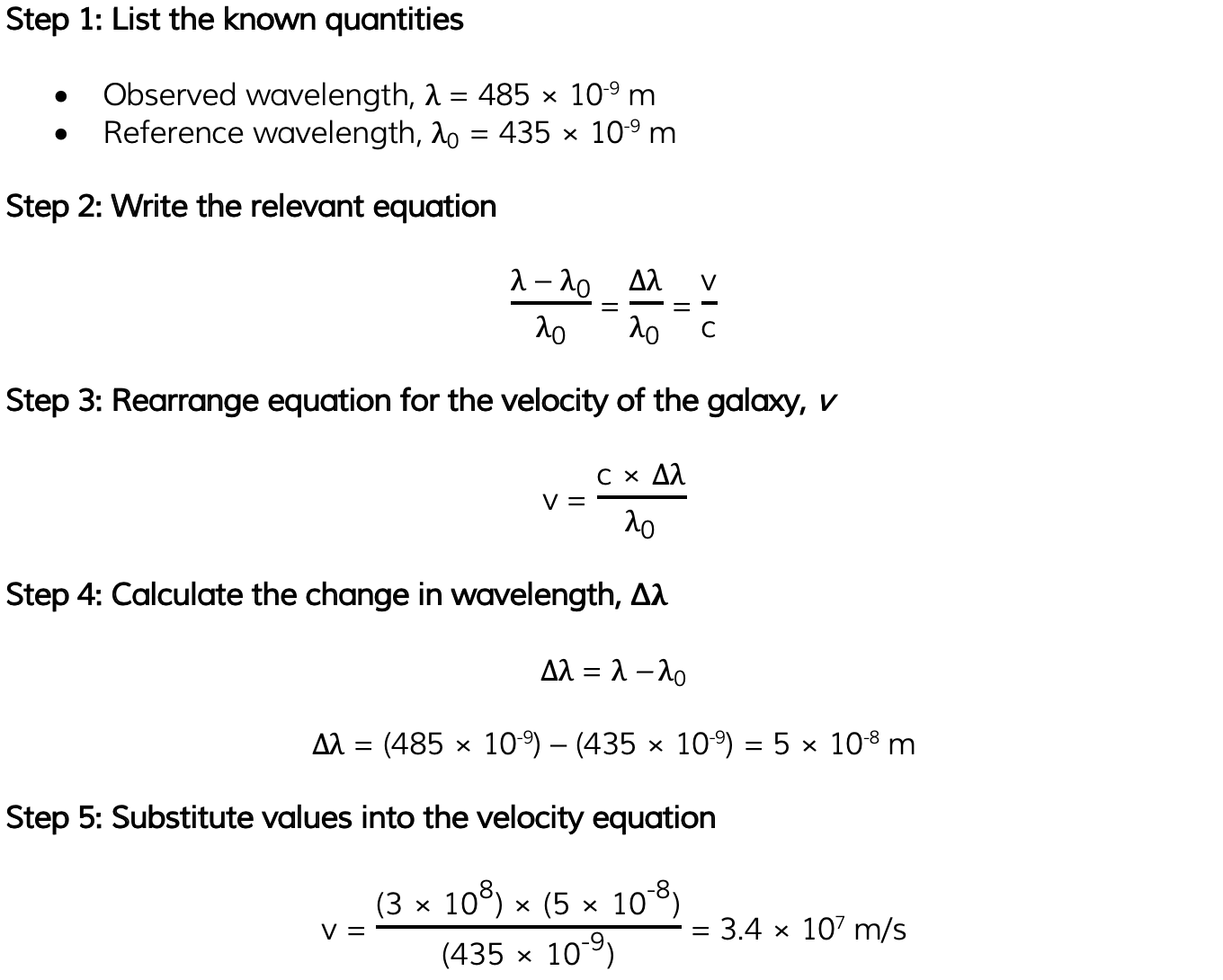# IB DP Physics: HL复习笔记9.5.3 The Doppler Equation

### The Doppler Equation

#### Calculating Doppler Shift

• When a source of sound waves moves relative to a stationary observer, the observed frequency can be calculated using the equation below: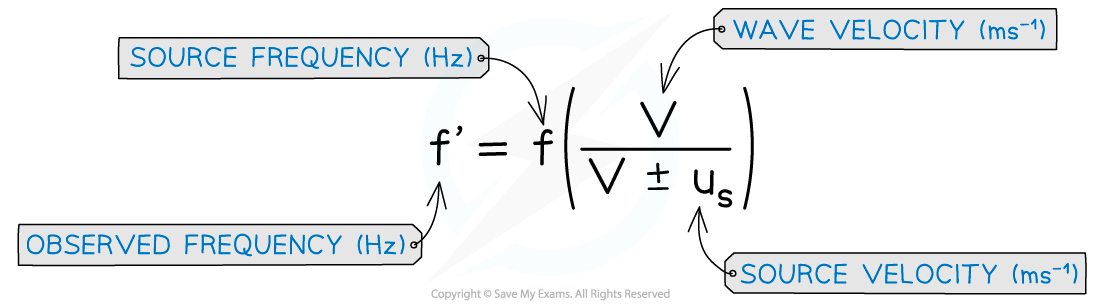Doppler shift equation for a moving source

• The wave velocity for sound waves is 340 ms-1
• The ± depends on whether the source is moving towards or away from the observer
• If the source is moving towards the observer, the denominator is v - us
• If the source is moving away from the observer, the denominator is v + us
• When a source of sound waves remains stationary, but the observer is moving relative to the source, the observed frequency can be calculated using the equation below: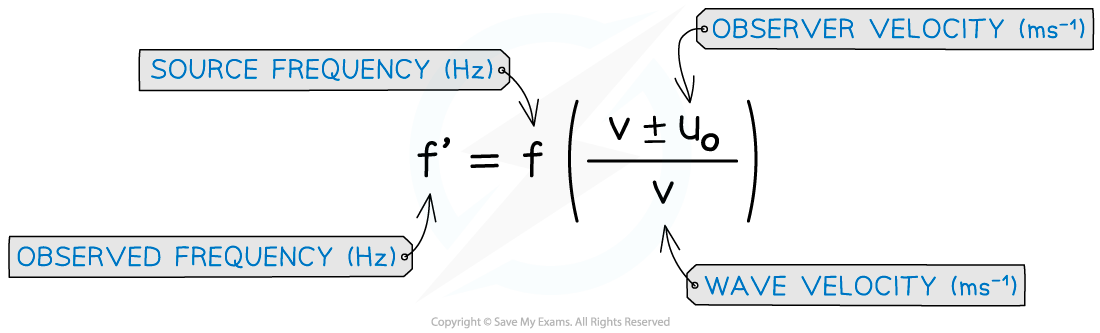Doppler shift equation for a moving observer

• The ± depends on whether the source is moving towards or away from the observer
• If the observer is moving towards the source, the numerator is v + uo
• If the observer is moving away from the source, the numerator is v − uo
• These equations can also be written in terms of wavelength
• For example, the equation for a moving source is shown below: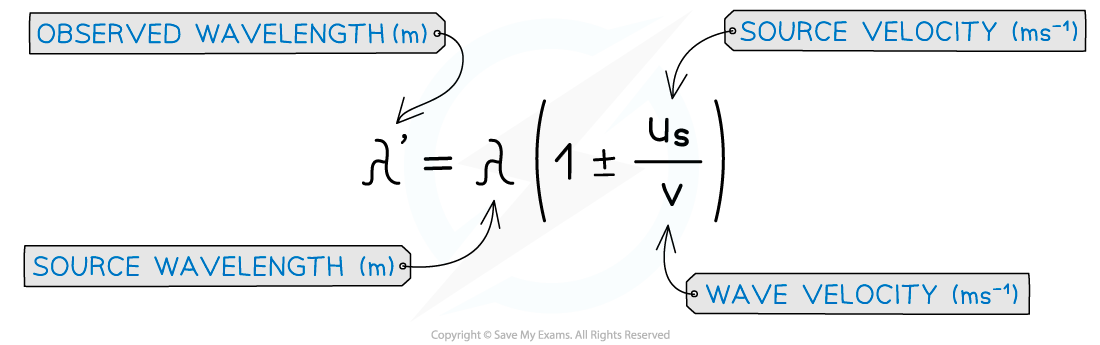Doppler shift equation for a moving source in terms of wavelength

####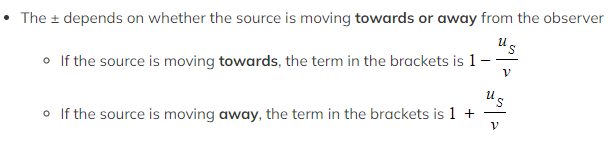Worked Example

A police car siren emits a sound wave with a frequency of 450 Hz. The car is traveling away from an observer at speed of 45 m s-1. The speed of sound is 340 m s-1. Which of the following is the frequency the observer hears?

A. 519 Hz               B. 483 Hz               C. 397 Hz               D. 358 Hz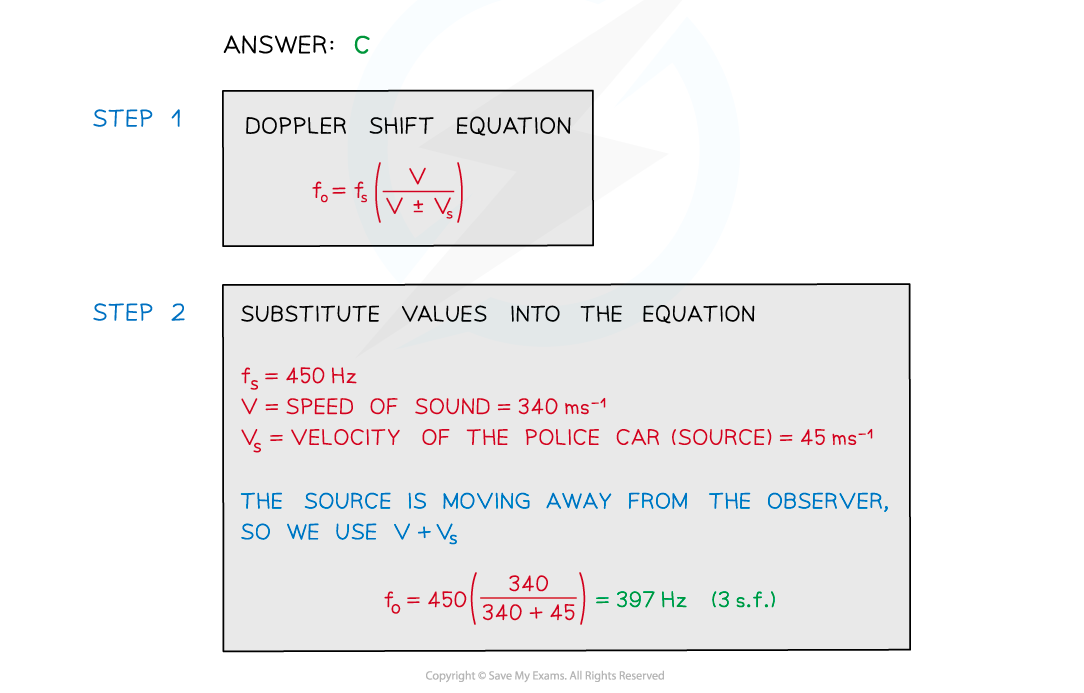#### Worked Example

A bank robbery has occurred and the alarm is sounding at a frequency of 3 kHz. The thief jumps into a car which accelerates and reaches a constant speed. As he drives away at a constant speed, the frequency decreases to 2.85 kHz. The speed of sound is 340 m s-1. Determine at what speed must he be driving away from the bank.

Step 1: List the known quantities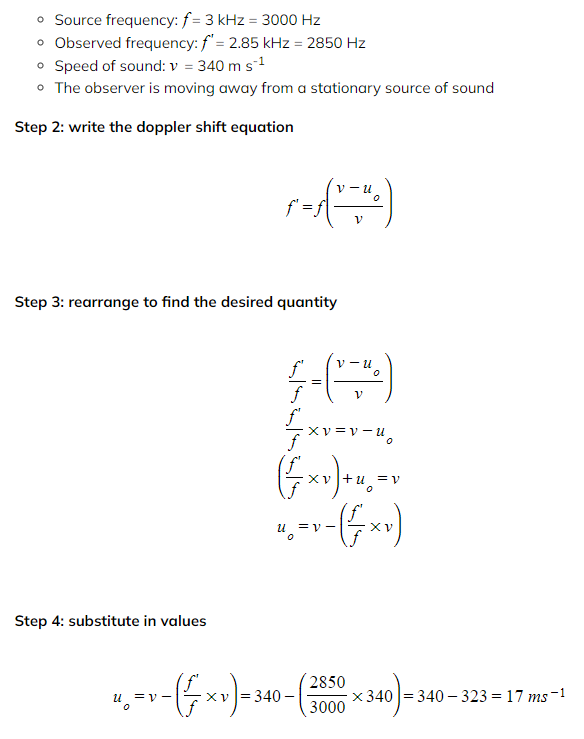Step 5: State final answer

• The bank robber must be driving away at a constant speed of 17 ms-1 based on the change in frequency heard

#### Calculating Doppler Shift of Light

• Doppler shift can be calculated with relation to a light emitting source
• For example, a galaxy moving towards or away from Earth
• Doppler shift for light is complicated, however if the speed of the observer or source is small (non-relativistic) compared to the speed of light, then this equation becomes simpler
• The Doppler shift for a light-emitting non-relativistic source is described using the equation:Doppler shift equation relating wavelength change for a moving source

• Where:
• Δf = change in frequency in Hertz (Hz)
• f0 = reference frequency in Hertz (Hz)
• λ = observed wavelength of the source in metres (m)
• λ0 = reference wavelength in metres (m)
• Δλ = change in wavelength in metres (m)
• v = velocity of a galaxy in metres per seconds (m/s)
• c = the speed of light in metres per second (m/s)
• This means that the change in wavelength, Δλ:

Δλ = λ – λ0

• This equation can be used to calculate the velocity of a galaxy if its wavelength can be measured and compared to a reference wavelength
• Since the fractions have the same units on the numerator (top number) and denominator (bottom number), the Doppler shift has no units

#### Worked Example

Light emitted from a star has a wavelength of 435 × 10-9 m. A distance galaxy emits the same light but has a wavelength of 485 × 10-9 m. Calculate the speed at which the galaxy is moving relative to Earth. The speed of light = 3 × 108 m/s.